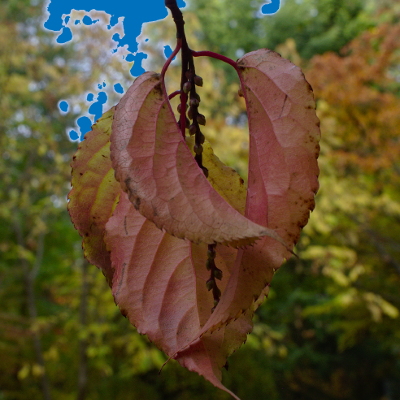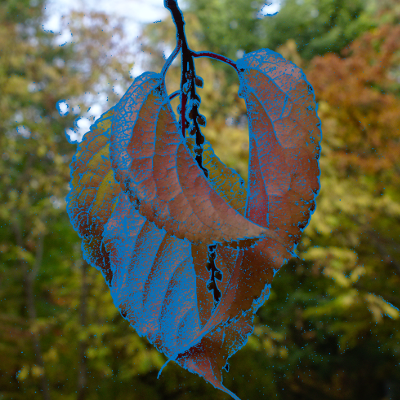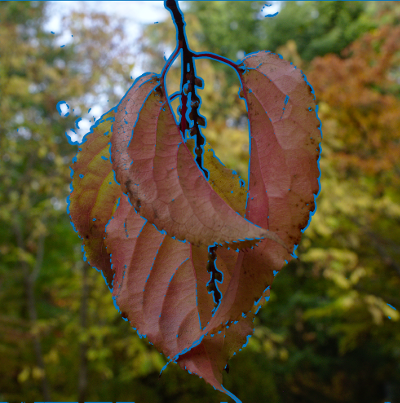UNB/ CS/ David Bremner/ teaching/ cs2613/ labs/ Lab 25

Thresholding: overexposure detection

Time
25 minutes
Activity
Small Groups

One common feature of photo editing software (or camera firmware) is to highlight overexposed or underexposed pixels. In this exercise we will use our solutions for `scale_layers` and `monochrome` from L24 in order to mark the pixels above a certain level.

Download the following image (make sure you download the actual full resolution image) and save it as `~/fcshome/cs2613/labs/L25/leaf.jpg`.Use the builtin function `find` to complete the `threshold` function to find all pixel positions with value higher than a given threshold. Note that this use of `find` depends on broadcasting. In all of the calls to `plot` in this lab, you may want to adjust `markersize` to better see the selected pixels (it depends a bit on screen resolution and image size).

```function [rows, cols] = threshold(in, lower=100)
[rows,cols] =
endfunction

%!test
%! A=[1,2,3; 4,5,6];
%!  [rows,cols]=threshold(A,4);
%!  assert(rows==[2;2]);
%!  assert(cols==[2;3]);

%!demo
%! monoleaf=monochrome(leaf);
%! [rows, cols] = threshold(monoleaf,200);
%! clf
%! hold on;
%! imshow(leaf);
%! plot(cols,rows,".","markersize",1);
```

The output of `demo threshold` should be something like the following.Time
15 minutes
Activity
Small Groups

In order to extract a description of a scene from an image, an early step is to detect the boundaries between various objects in the scene via edge detection. There are many different methods, but one approach is to find pixels where the gradient is particularly high. Use the `monochrome` and `normgrad` functions from L24 to complete the following script.

```leaf=imread("leaf.jpg");
monoleaf=
ng =
[rows, cols] = threshold(ng, 8 );

clf
hold on;
imshow(leaf);
plot(cols,rows,".","markersize",1);
```

The output of this script should be something like the following; it's interesting as far as detecting texture, but not very helpful for dividing the scene into objects. There's also a fair amount of isolated "edge" pixels in the background. Both of these problems can be addressed via smoothing in the next section.Convolution and smoothing I

Time
10 minutes
Activity
Demo

In order to smooth out some of the noise (or just isolated bright pixels), we can replace the value of each pixel with a weighted average of it's neighbourhood. The simplest approach is the so called box blur, where each pixel in the neighbourhood is added up, and the result divided by the number of pixels. The Octave function `conv2(A,B)` provides an easy way to apply a convolution or kernel) to a matrix. This just means that `B` specifies the weights in averaging the neighbourhood.

Fill in the appropriate matrix (formula) `B` to make the following smoothing demo work.

```A = [0,0,0,0,0,1;
0,1,1,1,0,0;
0,1,1,1,0,1;
0,1,1,1,1,0;
0,1,1,1,1,0;
0,0,0,0,0,0];

for j=2:12
B=
AB = conv2(A,B);
imshow(AB,[]);
pause(1);
endfor
```

Convolution and Smoothing II

Time
25 minutes
Activity
Small groups

Generalized the demo above to make a smoothing function. For variety we're going to use the Paris image image from last lab for the demo.

```function S=smooth(in, side=3)
S =
endfunction

%!test
%! A = [0,0,0,1;
%!      1,1,0,0;
%!      1,1,0,1;
%!      1,1,1,0];
%! B= [0.50, 0.25, 0.25, 0.25;
%!     1.00, 0.50, 0.25, 0.25;
%!     1.00, 0.75, 0.50, 0.25;
%!     0.50, 0.50, 0.25, 0.00];
%! assert(smooth(A,2),B,eps);

%!demo
%! monoparis=monochrome(paris,[1/3,1/3,1/3]);
%! imshow(monoparis,[])
%! pause(1);
%! imshow(smooth(monoparis),[])
%! pause(1);
%! imshow(smooth(monoparis,10),[])
%! pause(1);
%! imshow(smooth(monoparis,20),[])
```

Use your new function to complete the following script. Adjust the smoothing and threshold parameters to approximate the image below.

```leaf=imread("leaf.jpg");
monoleaf=monochrome(leaf);
smoothed=
[rows, cols] =

clf
hold on;
imshow(leaf,[]);
plot(cols,rows,".","markersize",1);
```Time
25 minutes
Activity
Small Groups

As you probably saw, the second argument to `conv2` can be much more general than just uniform weights. In particular some of the weights can be negative, e.g. to approximate a `gradient` calculation.

One example is the Soebel Operator which combines gradiant and smoothing operations.

Refering to the Wikipedia page, complete the function to calculuate the Soebel operator. You will also need to define function `norm2`, which can be copied from the definition of `normgrad` (without the gradient part).

```function [Gx,Gy]=soebel(in)

Gx =
Gy =
endfunction

%!demo